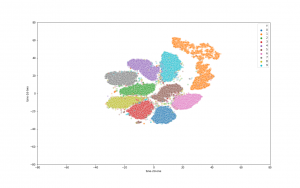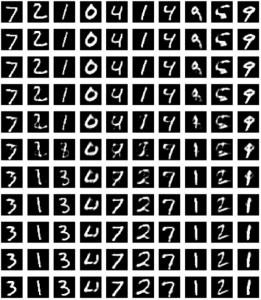# Introduction

Autoencoders provide a powerful framework for learning compressed representations by encoding all of the information needed to reconstruct a data point in a latent code. In some cases, autoencoders can “interpolate”: By decoding the convex combination of the latent codes for two data points, the autoencoder can produce an output that semantically mixes characteristics from the data points. In this paper, we propose a regularization procedure that encourages interpolated outputs to appear more realistic by fooling a critical network that has been trained to recover the mixing coefficient from interpolated data. We then develop a simple benchmark task where we can quantitatively measure the extent to which various autoencoders can interpolate and show that our regularizer dramatically improves interpolation in this setting. We also demonstrate empirically that our regularizer produces latent codes which are more effective on downstream tasks, suggesting a possible link between interpolation abilities and learning useful representations. – 

The idea comes from the paper “Implementation from the paper: Understanding and Improving Interpolation in Autoencoders via an Adversarial Regularizer” (https://arxiv.org/abs/1807.07543), also known as ACAI framework.

Today I will walk through the implementation of this fantastic idea. The implementation is based on tensorflow 2.0 and python 3.6. Let’s start!

# Implementation

First, we need to import some dependency packages.

```import tensorflow as tf
from tensorflow import keras
from tensorflow.keras.datasets import mnist
from tensorflow.keras.layers import Input, Dense, Reshape, Flatten, Dropout, multiply, GaussianNoise
from tensorflow.keras.layers import BatchNormalization, Activation, Embedding, ZeroPadding2D
from tensorflow.keras.layers import MaxPooling2D
from tensorflow.keras.layers import LeakyReLU
from tensorflow.keras.layers import UpSampling2D, Conv2D, Reshape
from tensorflow.keras.layers import Lambda
from tensorflow.keras.models import Sequential, Model
from tensorflow.keras import losses
from tensorflow.keras.utils import to_categorical
from tensorflow.keras.datasets import mnist
import keras.backend as K

import matplotlib.pyplot as plt

import numpy as np
import tqdm
import os

import io
from PIL
import Image
from sklearn.decomposition import PCA
# from sklearn.manifold import TSNE
import seaborn as sns
import pandas as pd
```

Next, we define the overall framework of ACAI, which composes of 3 parts: encoder, decoder and discriminator (also called as critic in the paper).

```class ACAI():
def __init__(self, img_shape=(28,28), latent_dim=32, disc_reg_coef=0.2, ae_reg_coef=0.5, dropout=0.0):
self.latent_dim = latent_dim
self.img_shape = img_shape
self.dropout = dropout
self.disc_reg_coef = disc_reg_coef
self.ae_reg_coef = ae_reg_coef
self.intitializer = tf.keras.initializers.VarianceScaling(
scale=0.2, mode='fan_in', distribution='truncated_normal')
self.initialize_models(self.img_shape, self.latent_dim)

def initialize_models(self, img_shape, latent_dim):
self.encoder = self.build_encoder(img_shape, latent_dim)
self.decoder = self.build_decoder(latent_dim, img_shape)
self.discriminator = self.build_discriminator(latent_dim, img_shape)

img = Input(shape=img_shape)
latent = self.encoder(img)
res_img = self.decoder(latent)

self.autoencoder = Model(img, res_img)
discri_out = self.discriminator(img)

def build_encoder(self, img_shape, latent_dim):
encoder = Sequential(name='encoder')

encoder.summary()
return encoder

def build_decoder(self, latent_dim, img_shape):
decoder = Sequential(name='decoder')

decoder.summary()
return decoder

def build_discriminator(self, latent_dim, img_shape):
discriminator = Sequential(name='discriminator')

discriminator.summary()
return discriminator```

Some utility functions for monitoring the results:

```def make_image_grid(imgs, shape, prefix, save_path, is_show=False):
# Find the implementation in below github repo

def flip_tensor(t):
# Find the implementation in below github repo

def plot_to_image(figure):
# Find the implementation in below github repo

def visualize_latent_space(x, labels, n_clusters, range_lim=(-80, 80), perplexity=40, is_save=False, save_path=None):
# Find the implementation in below github repo```

Next, we define the training worker, which is called at each epoch:

```@tf.function
def train_on_batch(x, y, model: ACAI):
# Randomzie interpolated coefficient alpha
alpha = tf.random.uniform((x.shape, 1), 0, 1)
alpha = 0.5 - tf.abs(alpha - 0.5)  # Make interval [0, 0.5]

# Constructs non-interpolated latent space and decoded input
latent = model.encoder(x, training=True)
res_x = model.decoder(latent, training=True)

ae_loss = tf.reduce_mean(tf.losses.mean_squared_error(tf.reshape(x, (x.shape, -1)), tf.reshape(res_x, (res_x.shape, -1))))

inp_latent = alpha * latent + (1 - alpha) * latent[::-1]
res_x_hat = model.decoder(inp_latent, training=False)

pred_alpha = model.discriminator(res_x_hat, training=True)
# pred_alpha = K.mean(pred_alpha, [1,2,3])
temp = model.discriminator(res_x + model.disc_reg_coef * (x - res_x), training=True)
# temp = K.mean(temp, [1,2,3])
disc_loss_term_1 = tf.reduce_mean(tf.square(pred_alpha - alpha))
disc_loss_term_2 = tf.reduce_mean(tf.square(temp))

reg_ae_loss = model.ae_reg_coef * tf.reduce_mean(tf.square(pred_alpha))

total_ae_loss = ae_loss + reg_ae_loss
total_d_loss = disc_loss_term_1 + disc_loss_term_2

return {
'res_ae_loss': ae_loss,
'reg_ae_loss': reg_ae_loss,
'disc_loss': disc_loss_term_1,
'reg_disc_loss': disc_loss_term_2

}```

Next, we need to define a main training function:

```def train(model: ACAI, x_train, y_train, x_test,
batch_size, epochs=1000, save_interval=200,
save_path='./images'):
n_epochs = tqdm.tqdm_notebook(range(epochs))
total_batches = x_train.shape // batch_size
if not os.path.exists(save_path):
os.makedirs(save_path)
for epoch in n_epochs:
offset = 0
losses = []
random_idx = np.random.randint(0, x_train.shape, x_train.shape)
for batch_iter in range(total_batches):
# Randomly choose each half batch
imgs = x_train[offset:offset + batch_size,::] if (batch_iter < (total_batches - 1)) else x_train[offset:,::]
offset += batch_size

loss = train_on_batch(imgs, None, model)
losses.append(loss)

avg_loss = avg_losses(losses)
# wandb.log({'losses': avg_loss})

if epoch % save_interval == 0 or (epoch == epochs - 1):
sampled_imgs = model.autoencoder(x_test[:100])
res_img = make_image_grid(sampled_imgs.numpy(), (28,28), str(epoch), save_path)

latent = model.encoder(x_train, training=False).numpy()
latent_space_img = visualize_latent_space(latent, y_train, 10, is_save=True, save_path=f'./latent_space/{epoch}.png')
# wandb.log({'res_test_img': [wandb.Image(res_img, caption="Reconstructed images")],
#            'latent_space': [wandb.Image(latent_space_img, caption="Latent space")]})```
```(x_train, y_train), (x_test, y_test) = mnist.load_data()
x_train = x_train.astype(np.float32) / 255.
x_test = x_test.astype(np.float32) / 255.
ann = ACAI(dropout=0.5)
train(model=ann,
x_train=x_train,
y_train=y_train,
x_test=x_test,
batch_size=x_train.shape//4,
epochs=2000,
save_interval=50,
save_path='./images')```

# Results

Some of the result from ACAI after finishing:

First is the visualization of MNIST dataset after encoded by the encoder, we can see that the cluster is well separated, and applying downstream tasks on latent space will lead to significant improvement in comparison to raw data (such as clustering, try KMeans and check it out yourself :D).Second is the visualization of interpolation power on latent space:

• Interpolation with alpha values in range [0,1.0] with step 0.1.
• 1st row and final rows are the source and destination images, respectively.
• Formula:
``````mix_latent = alpha * src_latent + (1 - alpha) * dst_latent
``````We can see that there is a very smooth morphing from the digits at the top row to the digits at the bottom row.

The whole running code is available at github (acai_notebook). It’s your time to play with the paper :D.

# Reference

 David Berthelot, Colin Raffel, Aurko Roy, and Ian Goodfellow. Understanding and improving interpolation in autoencoders via an adversarial regularizer, 2018.

# Hiring Data Scientist / Engineer

We are looking for Data Scientist and Engineer.